Topics: AI | Data Science | Data Visualization | Deep Learning | Machine Learning | NLP | Python | R | Statistics

KDnuggets Home » News » 2019 » Jun » Tutorials, Overviews » Make your Data Talk! ( 19:n25 )

Matplotlib and Seaborn are two of the most powerful and popular data visualization libraries in Python. Read on to learn how to create some of the most frequently used graphs and charts using Matplotlib and Seaborn.

(Tip #9)

16) You can draw vertical or horizontal lines inn plot by using functions `plt.axhline`, `plt.axvline`, or `ax.axline`, `ax.axvline`.

H] Be a good storyteller, and convey your findings through a story in a way that is easily understood by masses and gets the message across.

```from matplotlib.pyplot import figure
figure(figsize=(10, 7))

vp = plt.violinplot(train_df['target'], vert=False, showmeans=True,
showmedians=True)

# Returns a dictionary with keys : ['bodies', 'cbars', 'cmaxes',
#                                   'cmeans', 'cmedians', 'cmins']
# Using these we can tinker with our plot:
vp['bodies'].set_edgecolor("k")
vp['bodies'].set_linewidth(2)
vp['bodies'].set_alpha(1.0)
vp['bodies'].set_zorder(10)

vp['cmeans'].set_linestyle(":")
vp['cmeans'].set_color("r")
vp['cmeans'].set_zorder(101)
vp['cmeans'].set_segments(np.array([[[2.06855817, 0.7], [2.06855817, 1.3]]]))```

vp['cmedians'].set_linestyle("--")
vp['cmedians'].set_color("orange")
vp['cmedians'].set_zorder(100)
vp['cmedians'].set_segments(np.array([[[1.797, 0.7], [1.797, 1.3]]]))

vp['cbars'].set_zorder(99)
vp['cbars'].set_color("k")
vp['cbars'].set_linewidth(0.5)

vp['cmaxes'].set_visible(False)
vp['cmins'].set_visible(False)

# Legend:
plt.legend(handles=[vp['bodies'], vp['cmeans'], vp['cmedians']],
labels=["Target", "Mean", "Median"], handlelength=5)
plt.title("Target Violin Plot")
plt.xlabel("Target")
plt.yticks([])
plt.grid(True, alpha=0.8)

plt.text(x, y, f"({train_df['target'].median()}) Median",
'alpha': 0.7}, zorder=12)
plt.text(x2, y2, f"Mean ({np.round(train_df['target'].mean(),3)})",
'alpha': 0.6}, zorder=11);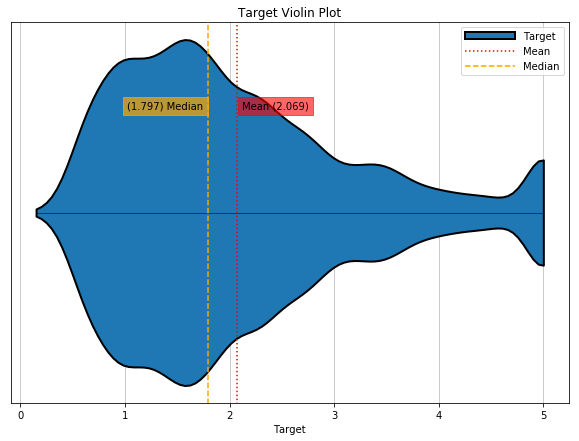Storytelling With Matplotlib (SWMat)

`TK Work in Progress...`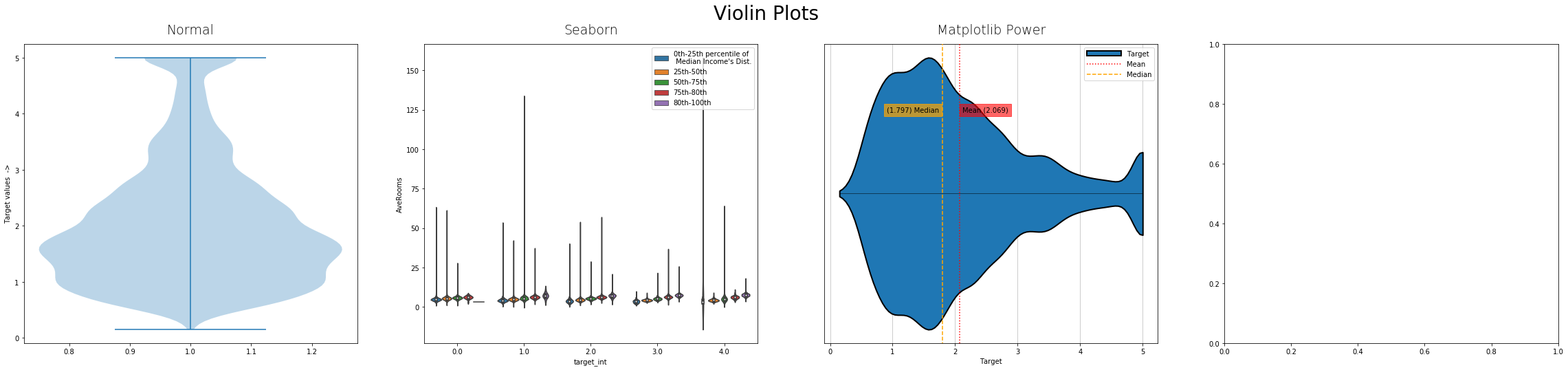1) Normal Matplotlib, 2) Seaborn, 3) Matplotlib Power, 4) Storytelling With Matplotlib

### 5. Multiple PlotsYou can make as many plots as you need either by using `plt.subplots` method or manually add `Axes`'s to figure by specifying their box coordinates, or by using `plt.GridSpec()` method. I.e.

1. Either by using: `fig, axess = plt.subplots(ncols=2, nrows=4)` and then you can draw in any one of these `Axes`'s by accessing them as `axess[col_num][row_rum]`, and then use any of `Axes` methods to draw in them.
2. Or by using `plt.axes()` method giving list of four percent values giving [left, bottom, width, height] of `Axes` to make in `figure`. For example: `plt.axes([0.1, 0.1, 0.65, 0.65)`.
3. Or by using `plt.GridSpec()` method. As `grid = plt.GridSpec(n_row, n_col)`. And now while making `Axes` by `plt.subplot()` method you can use this `grid` as an 2D array to select how many and which grids to use for making current, one, `Axes`. For example `plt.subplot(grid[0,:])` will select whole first row as one `Axes`. If you want you can leave some of them too.
```plt.figure(1, figsize=(10, 8))
plt.suptitle("Hist-Distribution", fontsize=18, y=1)

# Now lets make some axes in this figure
axScatter = plt.axes([0.1, 0.1, 0.65, 0.65])
# [left, bottom, width, height] in percent values
axHistx = plt.axes([0.1, 0.755, 0.65, 0.2])
axHisty = plt.axes([0.755, 0.1, 0.2, 0.65])

axHistx.set_xticks([])
axHistx.set_yticks([])
axHisty.set_xticks([])
axHisty.set_yticks([])
axHistx.set_frame_on(False)
axHisty.set_frame_on(False)
axScatter.set_xlabel("MedInc  ->")
axScatter.set_ylabel("Population  ->")

# Lets plot in these axes:
axScatter.scatter(x, y, edgecolors='w')
axHistx.hist(x, bins=30, ec='w', density=True, alpha=0.7)
axHisty.hist(y, bins=60, ec='w', density=True, alpha=0.7,
orientation='horizontal')
axHistx.set_ylabel("")

axScatter.annotate("Probably an outlier", xy=(2.6, 35500),
xytext=(7, 28000),
arrowprops={'arrowstyle':'->'},
0.4, 'edgecolor':'orange'});

```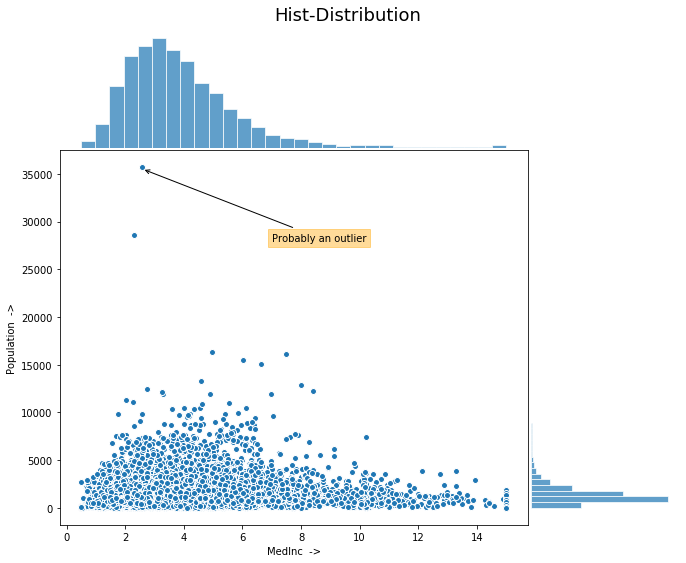(Tip #10)

17)` seaborn` has its own objects for grids/multiplots namely `Facet Grid`,`Pair Grid` and ` Joint Grid `. They have some methods like `.map`,`.map_diag`, `.map_upper`, `.map_lower` etc that you can look into to draw plots in those locations only in 2D grid.

I] Read the book “Storytelling with data” by Cole N. Knaflic. Its a great read covering every aspect with examples by a well known Data Communicator.

```from matplotlib.pyplot import figure
figure(figsize=(10, 8))

sns.jointplot(x, y);

```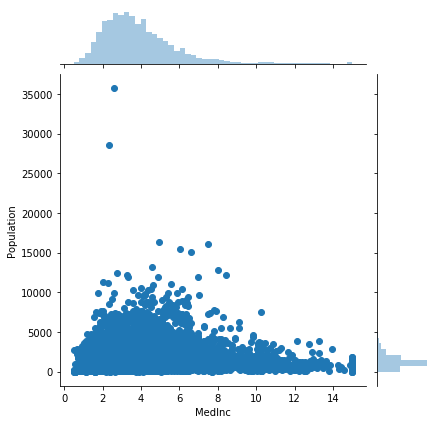### 6. Interactive PlotsBy default Interactive plotting in `matplotlib` is turned off. That means that plot will be shown to you only after you have given your final `plt` command or you used a command that triggers `plt.draw` like `plt.show()`. You can turn on interactive plotting by `ion()` function and turn it off by `ioff()` function. By turning it on every `plt` function will trigger `plt.draw`.

In modern Jupyter Notebook/IPython world there is one magic command to turn on Interactive/Animation feature in these notebooks, and that is `%matplotlib notebook` and to turn it off you can use magic command `%matplotlib inline` before using any of your `plt` functions.

`matplotlib` works with a number of user interface toolkits (wxpython, tkinter, qt4, gtk, and macosx) to show interactive plots. For these interactive plots `matplotlib` uses `event`'s and event handler/manager (`fig.canvas.mpl_connect`) to capture some event by mouse or keyboard.

This event manager is used to connect some in-built event-type-looker to a custom function which will be evoked if that particular type of event happens.

There are many events available like ‘ button_press_event’, ‘button_release_event’, ‘ draw_event’, ‘ resize_event’, ‘ figure_enter_event’, etc. which you can connect to like `fig.canvas.mpl_connect(event_name, func)`.

For above example if `event_name` event happens, all related data to that event will be sent to your function `func` where you should have coded something to use that data provided. This event data contains information like x and y position, x and y data coordinates, weather click was made inside `Axes` or not, etc. if they are relevant for your event type `event_name`.

```%matplotlib notebook
# Example from matplotlib Docs

class LineBuilder:
def __init__(self, line):
self.line = line
self.xs = list(line.get_xdata())
self.ys = list(line.get_ydata())
self.cid = line.figure.\
canvas.mpl_connect('button_press_event', self)

def __call__(self, event):
print('click', event)
if event.inaxes!=self.line.axes: return
self.xs.append(event.xdata)
self.ys.append(event.ydata)
self.line.set_data(self.xs, self.ys)
self.line.figure.canvas.draw()

fig = plt.figure()
ax.set_title('click to build line segments')
line, = ax.plot(, )  # empty line
linebuilder = LineBuilder(line)

# It worked with a class because this class has a __call__
# method.

```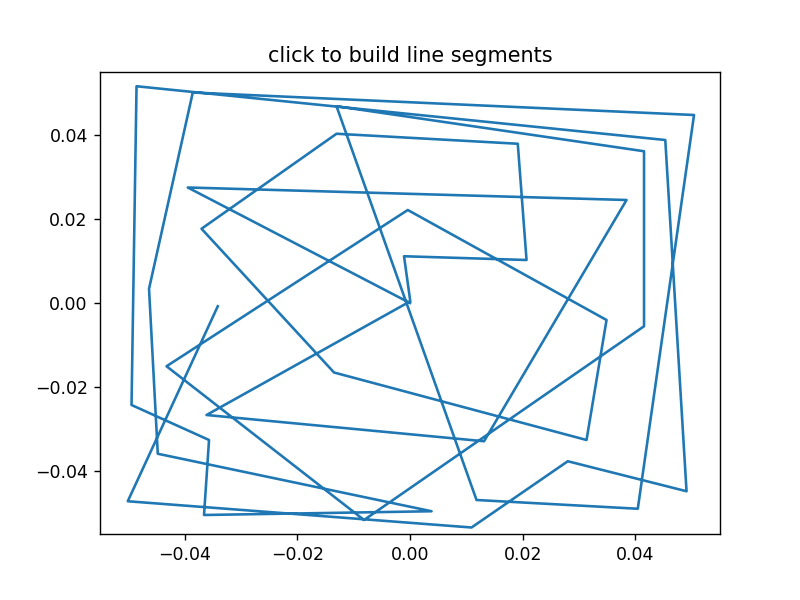Random lines drawn using above code (by consecutive clicking)

### 7. OthersPhoto by rawpixel on Unsplash

3D Plots:

3D plots of `matplotlib` are not in usual lib. It is in `mpl_toolkits` as `matplotlib` started with only 2D plots and later on it added 3D plots in `mpl_toolkits`. You can import it as `from mpl_toolkits import mplot3d`.

After importing you can make any `Axes` 3D axes by passing `projection='3d'` to any `Axes` initializer/maker function.

`ax = plt.gca(projection='3d') # Initialize...`

```# Data for a three-dimensional line
zline = np.linspace(0, 15, 1000)
xline = np.sin(zline)
yline = np.cos(zline)
ax.plot3D(xline, yline, zline, 'gray')```

```# Data for three-dimensional scattered points
zdata = 15 * np.random.random(100)
xdata = np.sin(zdata) + 0.1 * np.random.randn(100)
ydata = np.cos(zdata) + 0.1 * np.random.randn(100)
ax.scatter3D(xdata, ydata, zdata, c=zdata, cmap='Greens');```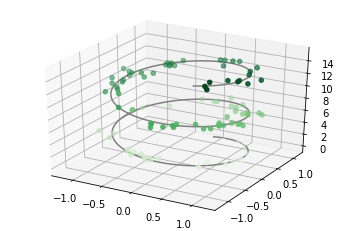(Tip #11)

18) You can look at 3D plots interactively by running `%matplotlib notebook` before your plotting functions.

There are many 3D plots available like `line`, `scatter`, `wireframe`, `surface` plot, `contour`, `bar` etc and even `subplot` is also available. You can also write on these plots with `text` function.

```# This import registers the 3D projection, but is otherwise unused.
from mpl_toolkits.mplot3d import Axes3D

# setup the figure and axes
plt.figure(figsize=(8, 6))
ax = plt.gca(projection='3d')

ax.bar3d(x, y, bottom, width, depth, top, shade=True)
ax.set_title('Bar Plot')
```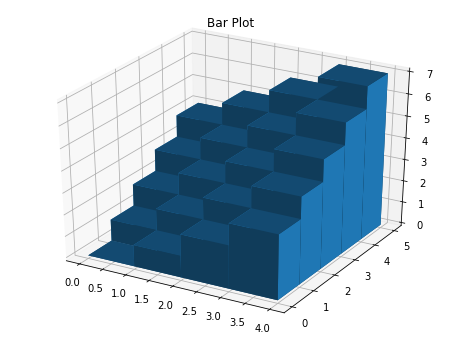Geographical Plots:

To plot Geographic plots with `matplotlib` you will have to install another package by `matplotlib` called `Basemap`. It is not easy to install, look for official instructions here, or you can use `conda` command if you have Anaconda installed: `conda install -c conda-forge basemap`, or if these too doesn’t work for you look here (specifically last comment).

```from mpl_toolkits.basemap import Basemap

m = Basemap()
m.drawcoastlines()

```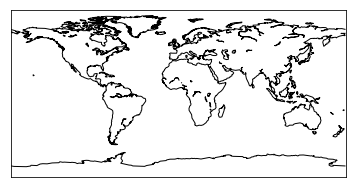You can actually use most of matplotlib’s original functions here like `text`, `plot`, `annotate`, `bar`, `contour`, `hexbin` and even 3D plots on these projections.

And it also has some functions related to geographic plots too like `streamplot`, `quiver` etc.

```m = Basemap(projection='ortho', lat_0=0, lon_0=0)
# There are a lot of projections available. Choose one you want. m.drawmapboundary(fill_color='aqua')
m.fillcontinents(color='coral',lake_color='aqua')
m.drawcoastlines()

x, y = map(0, 0) # Converts lat, lon to plot's x, y coordinates.

m.plot(x, y, marker='D',color='m')

```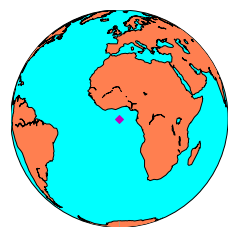```# llcrnr: lower left corner; urcrnr: upper right corner
m = Basemap(llcrnrlon=-10.5, llcrnrlat=33, urcrnrlon=10.,
urcrnrlat=46., resolution='l', projection='cass',
lat_0 = 39.5, lon_0 = 0.)
m.bluemarble()
m.drawcoastlines()```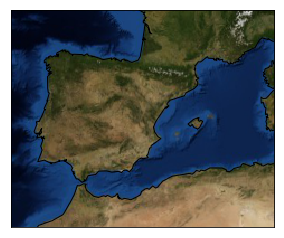```from mpl_toolkits.mplot3d import Axes3D

m = Basemap(llcrnrlon=-125, llcrnrlat=27, urcrnrlon=-113,
urcrnrlat=43, resolution='i')

fig = plt.figure(figsize=(20, 15))
ax = Axes3D(fig)

ax.set_axis_off()
ax.azim = 270 # Azimuth angle
ax.dist = 6   # Distance of eye-viewing point fro object point

x, y = m(x, y)
ax.bar3d(x, y, np.zeros(len(x)), 30, 30, np.ones(len(x))/10,
color=colors, alpha=0.8)
```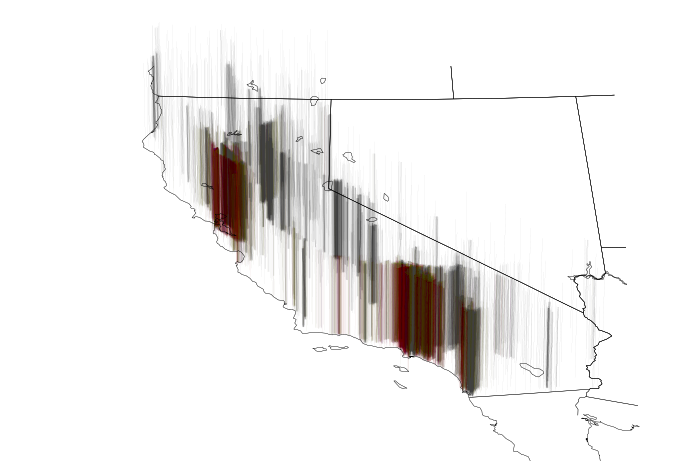‘Target’ distribution (red -> high) in California. [From above used California Dataset]

Word Cloud Plot:

Word Clouds are used in Natural Language Processing (NLP), showing words having most frequencies, having size depending on their frequency, within some boundary which can be a cloud or not. It plots relative frequency difference between words in data as relative size of their font. It is also easy, most of the times, to get words with highest frequencies just by looking at Word Clouds. But still it is an interesting way to convey data as it is well perceived and easily understood.

There is a python package `wordcloud` which you can install using `pip` as `pip install wordcloud`.

You can first set some properties of `WordCloud` (like setting a cloud shape using `mask` parameter, specifying `max_words`, specifying `stopwords` etc.) and then generate cloud with specified properties for given text data.

```from wordcloud import WordCloud, STOPWORDS

# Create and generate a word cloud image:
wordcloud = WordCloud()\    # Use default properties
.generate(text)

# Display the generated image:
plt.imshow(wordcloud, interpolation='bilinear')
plt.axis("off")
```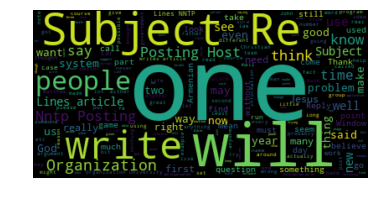```from PIL import Image
mask = np.array(Image.open("jour.jpg")) # Searched "journalism
# images...
stopwords = set(STOPWORDS)

stopwords=stopwords)

# Generate a wordcloud
wc.generate(text)

# show
plt.figure(figsize=[20,10])
plt.imshow(wc, interpolation='bilinear')
plt.axis("off")
plt.show()

```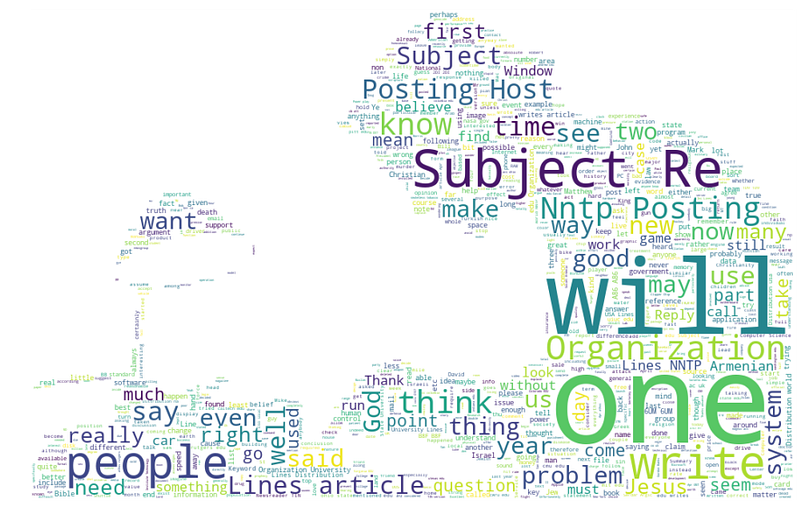Animations:

You can easily make animations using `matplotlib` using one of these two classes:

1. `FuncAnimatin`: makes an animation by repeatedly calling a function `func`.
2. `ArtistAnimation`: Animation using a fixed set of `Artist` objects.

(Tip #12)

19) Always keep a reference to instance object `Animation`, otherwise it will be garbage collected.

20) To save an animation to disk use one of `Animation.save` or `Animation.to_html5_video` methods.

21) You can speed up/optimize your animation’s drawing by using parameter `blit` set to `True`. But if `blit=True` you will have to return an iterable of artists to be redrawn by `init_func`.

In `FuncAnimation` you need to pass atleast current `fig` and a function which will be called for each frame. Other than that you should also look into parameters `frames` (iterable, int, generator , None; source of data to pass to `func` and each frame of animation), `init_func` (function used to draw a clear frame, otherwise first frame from `frames` is used), and `blit` (weather to use blitting or not).

```%matplotlib notebook

fig, ax = plt.subplots()
xdata, ydata = [], []
ln, = plt.plot([], [], 'ro')

def init():
ax.set_xlim(0, 2*np.pi)
ax.set_ylim(-1, 1)
return ln,

def update(frame):
xdata.append(frame)
ydata.append(np.sin(frame))
ln.set_data(xdata, ydata)
return ln,
# Always keep reference to `Animation` obj
ani = FuncAnimation(fig, update, frames=np.linspace(0, 2*np.pi,
128), init_func=init, blit=True)

```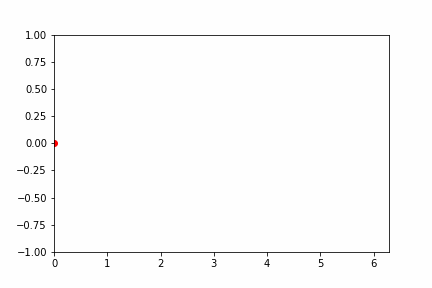1. Storytelling With Data — Cole N. Knaflic (Great book on how to Communicate Data using graphs/charts by a well known Data Communicator)
2. Python Data Science HandBook — Jake VanderPlas
3. Embedding Matplotlib Animations in Jupyter as Interactive JavaScript Widgets — Louis Tiao
4. Generating WordClouds in Python — Duong Vu
5. Basemap Tutorial

### 9. References

Suggestions and reviews are welcome. Thank you for reading!

Bio: Puneet Grover is a machine learning enthusiast.

Original. Reposted with permission.

Related:

Top Stories Past 30 Days
Most SharedGet KDnuggets, a leading newsletter on AI, Data Science, and Machine Learning